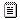Forums

## Slicing Implementation

General discussion about Cobra. Releases and general news will also be posted here.
Feel free to ask questions or just say "Hello".

### Slicing Implementation

Having noticed that Cobra had slicing syntax for lists, strings, arrays, etc.. that was not fully implemented, I went ahead and tried to remedy that.Implementation_for_Slicing.patch

This implementation uses the existing syntax "[start:stop:step]" where any of the three can be omitted and will be assumed based on the others. This assumption works like this:

• [start:stop] -> step is assumed to be 1 if start < stop or -1 if start > stop
• [start:::step] -> stop is assumed to be the beginning of the list if step < 0 or the end if step > 0
• [:stop:step] -> start is assumed to be the beginning of the list if step > 0 or the end if step < 0
• [::step] -> start and stop are assumed identically to the cases where they are individually omitted
• [::] -> this is just a copy of the list
• [start:] -> step is assumed to be 1 and stop is the end of the list
• [:stop] -> step is assumed to be 1 and start is the beginning of the list

NOTE: the example with "[start:::step]" is currently correct as for some reason the parser needs three colons.
Ourous

Posts: 10